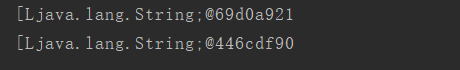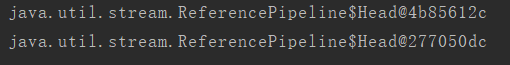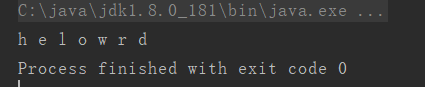# java8 实战读书笔记：初识 Stream、流的基本操作，nginx 架构原理

• 2021 年 11 月 10 日
• 本文字数：4696 字

阅读完需：约 15 分钟

<R> Stream<R> map(Function<? super T, ? extends R> mapper)

IntStream mapToInt(ToIntFunction<? super T> mapper)

LongStream mapToLong(ToLongFunction<? super T> mapper)

DoubleStream mapToDouble(ToDoubleFunction<? super T> mapper)

public static void test_flat_map() {

String[] strArr = new String[] {"hello", "world"};

List<String> strList = Arrays.asList(strArr);

strList.stream().map( s -> s.split(""))

.distinct().forEach(System.out::println);

}public static void test_flat_map() {

String[] strArr = new String[] {"hello", "world"};

List<String> strList = Arrays.asList(strArr);

strList.stream().map( s -> s.split(""))

.map(Arrays::stream)

.distinct().forEach(System.out::println);

}public static void test_flat_map() {

String[] strArr = new String[] {"hello", "world"};

List<String> strList = Arrays.asList(strArr);

strList.stream().map( s -> s.split(""))

.map(Arrays::stream)

.forEach( (Stream<String> s) -> {

System.out.println("\n --start---");

s.forEach(a -> System.out.print(a + " "));

System.out.println("\n --end---");

} );

}

public static void test_flat_map() {

String[] strArr = new String[] {"hello", "world"};

List<String> strList = Arrays.asList(strArr);

strList.stream().map( s -> s.split(""))

.flatMap(Arrays::stream)

.distinct().forEach( a -> System.out.print(a +" "));

}2.3 查找和匹配

Stream API 提供了 allMatch、anyMatch、noneMatch、findFirst 和 findAny 方法来实现对流中数据的匹配与查找。

2.3.1 allMatch

boolean allMatch(Predicate<? super T> predicate);

boolean result = a.stream().allMatch( a -> a % 2 == 0 )； // 将返回 false。

2.3.2 anyMatch

boolean anyMatch(Predicate<? super T> predicate)

boolean result = a.stream().anyMatch( a -> a % 2 == 0 )； // 将返回 true。

2.3.3 noneMatch

boolean noneMatch(Predicate<? super T> predicate);

boolean result = a.stream().noneMatch( a -> a % 2 == 1 )； // 将返回 true。

2.3.4 findFirst

Optional<T> findFirst();

public static void test_find_first(List<Dish> menu) {

// 这个方法表示，Optional 中包含 Dish 对象，则执行里面的代码，否则什么事不干，是不是比判断是否为 null 更友好

dish.ifPresent(a -> System.out.println(a.getName()));

}

2.3.5 findAny

Optional<T> findAny();

2.4 reduce

reduce 归约，看过大数据的人用过会非常敏感，目前的 java8 的流操作是不是有点 map-reduce 的味道，归约，就是对流中所有的元素进行统计分析，归约成一个数值。

T reduce(T identity, BinaryOperator<T> accumulator)

• T identity：累积器的初始值。

• BinaryOperator< T> accumulator：累积函数。BinaryOperator< T> extend BiFunction<T, U, R>。BinaryOperator 的函数式表示，接受两个 T 类型的入参，返回 T 类型的返回值。

Optional<T> reduce(BinaryOperator<T> accumulator);

<U> U reduce(U identity, BiFunction<U, ? super T, U> accumulator, BinaryOperator<U> combiner);

• U identity：累积函数的初始值。

• BiFunction<U, ? super T, U> accumulator：累积器函数，对流中的元素使用该累积器进行归约，在具体执行时 accumulator.apply( identity, 第二个参数的类型不做限制 )，只要最终返回 U 即可。

• BinaryOperator< U> combiner：组合器。对累积器的结果进行组合，因为归约 reduce，java 流计算内部使用了 fork-join 框架，会对流的中的元素使用并行累积，每个线程处理流中一部分数据，最后对结果进行组合，得出最终的值。

List<Integer> goodsNumber = Arrays.asList( 3, 5, 8, 4, 2, 13 );

java7 之前的示例：

int sum = 0;

for(Integer i : goodsNumber) {

sum += i;// sum = sum + i;

}

System.out.println("sum:" + sum);

public static void test_reduce() {

List<Integer> goodsNumber = Arrays.asList( 3, 5, 8, 4, 2, 13 );

int sum =

《Android学习笔记总结+最新移动架构视频+大厂安卓面试真题+项目实战源码讲义》浏览器打开：qq.cn.hn/FTe 免费领取

goodsNumber.stream().reduce(0, (a,b) -> a + b);

//这里也可以写成这样：

// int sum = goodsNumber.stream().reduce(0, Integer::sum);

System.out.println(sum);

}

public static void test_reduce_combiner() {

/** 初始化待操作的流 */

List<Integer> nums = new ArrayList<>();

int s = 0;

for(int i = 0; i < 200; i ++) {

s = s + i;

}

// 对流进行归并，求和,这里使用了流的并行执行版本 parallelStream，内部使用 Fork-Join 框架多线程并行执行，

// 关于流的内部高级特性，后续再进行深入，目前先以掌握其用法为主。

int sum2 = nums.parallelStream().reduce(0,Integer::sum, Integer::sum);

System.out.println("和为：" + sum2);

// 下面给出上述版本的 debug 版本。

// 累积器执行的次数

AtomicInteger accumulatorCount = new AtomicInteger(0);

// 组合器执行的次数（其实就是内部并行度）

AtomicInteger combinerCount = new AtomicInteger(0);

int sum = nums.parallelStream().reduce(0,(a,b) -> {

accumulatorCount.incrementAndGet();

return a + b;

}, (c,d) -> {

combinerCount.incrementAndGet();

return c+d;

});

System.out.println("accumulatorCount:" + accumulatorCount.get());

System.out.println("combinerCountCount:" + combinerCount.get());

}

1、filter

• 函数功能：过滤

• 操作类型：中间操作

• 返回类型：Stream

• 函数式接口：Predicate

• 函数描述符：T -> boolean

2、distinct

• 函数功能：去重

• 操作类型：中间操作

• 返回类型：Stream

3、skip

• 函数功能：跳过 n 个元素

• 操作类型：中间操作

• 返回类型：Stream

• 接受参数：long

4、limit

• 函数功能：截断流，值返回前 n 个元素的流

• 操作类型：中间操作

• 返回类型：Stream

• 接受参数：long

5、map

• 函数功能：映射

• 操作类型：中间操作

• 返回类型：Stream

• 函数式接口：Function<T,R>

• 函数描述符：T -> R

6、flatMap

• 函数功能：扁平化流，将多个流合并成一个流

• 操作类型：中间操作

• 返回类型：Stream

• 函数式接口：Function<T, Stream>

• 函数描述符：T -> Stream

7、sorted

• 函数功能：排序

• 操作类型：中间操作

• 返回类型：Stream

• 函数式接口：Comparator

• 函数描述符：(T,T) -> int

8、anyMatch

• 函数功能：流中任意一个匹配则返回 true

• 操作类型：终端操作

• 返回类型：boolean

• 函数式接口：Predicate

• 函数描述符：T -> boolean

9、allMatch

• 函数功能：流中全部元素匹配则返回 true

• 操作类型：终端操作

• 返回类型：boolean

• 函数式接口：Predicate

• 函数描述符：T -> boolean

10、 noneMatch

• 函数功能：流中所有元素都不匹配则返回 true

• 操作类型：终端操作

• 返回类型：boolean

• 函数式接口：Predicate

• 函数描述符：T -> boolean

11、findAny

• 函数功能：从流中任意返回一个元素

• 操作类型：终端操作

• 返回类型：Optional

12、findFirst

• 函数功能：返回流中第一个元素

• 操作类型：终端操作

• 返回类型：Optional

13、forEach

• 函数功能：遍历流

• 操作类型：终端操作

• 返回类型：void

• 函数式接口：Consumer

• 函数描述符：T -> void

14、collect

• 函数功能：将流进行转换

• 操作类型：终端操作

• 返回类型：R

• 函数式接口：Collector<T,A,R>

15、reduce

• 函数功能：规约流

• 操作类型：终端操作

• 返回类型：Optional

• 函数式接口：BinaryOperator

• 函数描述符：(T,T) -> T

16、count

• 函数功能：返回流中总元素个数

• 操作类型：终端操作

• 返回类型：long

## 评论